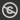Science, Maths & Technology
Free course

# Introduction to differential equations## Course reviews

Differential equations are any equations that include derivatives and arise in many situations. This free course, Introduction to differential equations, considers three types of first-order differential equations. Section 1 introduces equations that can be solved by direct integration and section 2 the method of separation of variables. Section 3 looks at applications of differential equations for solving real world problems. Section 4 introduces the integrating factor method for solving linear differential equations. The final two sections summarise and revise the methods introduced in the previous sections and describe various other approaches to finding solutions of first-order differential equations and to understanding the behaviour of the solutions.

## Course learning outcomes

After studying this course, you should be able to:

• recognise differential equations that can be solved by each of the three methods – direct integration, separation of variables and integrating factor method – and use the appropriate method to solve them
• use an initial condition to find a particular solution of a differential equation, given a general solution
• check a solution of a differential equation in explicit or implicit form, by substituting it into the differential equation
• understand the terms 'exponential growth/decay', 'proportionate growth rate' and 'doubling/halving time' when applied to population models, and the terms 'exponential decay', 'decay constant' and 'half-life' when applied to radioactivity
• solve problems involving exponential growth and decay.

First Published: 27/09/2017

Updated: 12/04/2018

Skip Rate and Review